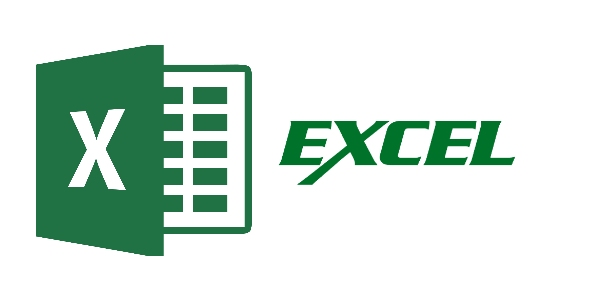# Excel Test - Fall 2012

30 Questions | Attempts: 141
ShareSettingsChoose the correct answer and click on it to select. Check your answers then submit to the teacher when you are finished.

• 1.
Excel's ___________ function allows you to add all of the numbers in a range of cells.
• A.

MIN

• B.

MAX

• C.

SUM

• D.

• 2.
The ___________ is a central location for managing workbooks with commands like Save, Print, and Open..
• A.

Ribbon

• B.

Office Button

• C.

Screen Tip

• D.

Share toolbar

• 3.
When typing formulas or functions into a cell, it is important to:
• A.

Begin the formula with an apostrophe

• B.

Lock the data into the cell by pressing enter when you're done

• C.

Type in lowercase

• D.

Split and Merge

• 4.
Excel opens a new workbook with _________ worksheets
• A.

One

• B.

Two

• C.

Three

• D.

Five

• 5.
To save a workbook using the shortcut keys, press
• A.

ALT + S

• B.

CTRL + S

• C.

SHIFT + S

• D.

CTRL + F2

• 6.
The ______________ is a blinking vertical line that indicates where the next typed character will appear.
• A.

Insertion point

• B.

Alignment tool

• C.

Left-justified

• D.

Right button

• 7.
When you create a formula in a cell that meets only specified conditions it is called a ...
• A.

Comma style formatting

• B.

Point mode

• C.

Conditional formula

• D.

Unlimited

• 8.
A saved workbook is referred to as a ____________.
• A.

Book

• B.

Workbook

• C.

Project

• D.

File

• 9.
The formula _____ multiplies the contents of cell B4 by 18.5
• A.

18.5 ^ B4

• B.

=18.5 * B4

• C.

=B4/18.5

• D.

=B4**18.5

• 10.
To alert Excel that you are entering a formula and not text, type a (n) ____________ before the formula
• A.

Ampersand (&)

• B.

Equal sign (=)

• C.

Number sign (#)

• D.

Asterisk (*)

• 11.
This is located on the Ribbon and is used to display the borders gallery
• A.

Active cell

• B.

Borders button

• C.

Name box

• D.

Forumula bar

• 12.
A user wishes to remove a spreadsheet from a workbook.  Which is the correct way to delete the tab?
• A.

Right click on the spreadsheet tab and select DELETE

• B.

• C.

Access the Office Button

• D.

Right click and rename

• 13.
• A.

• B.

Office Button

• C.

• D.

Name box

• 14.
The numbers on the left side of the Excel grid.
• A.

• B.

• C.

• D.

Footers

• 15.
The one into which you enter data and has a heavy border around it
• A.

Active cell

• B.

Cell name

• C.

Range

• D.

Worksheet

• 16.
The basic unit of a worksheet into which you enter data is called a ______________.
• A.

Cell

• B.

Box

• C.

Range

• D.

Gridlines

• 17.
Displays the lowest value for a range of cells
• A.

MAX

• B.

MIN

• C.

SUM

• D.

LOW

• 18.
The small black square located in the lower-right corner of the heavy border around the active cell
• A.

Name box

• B.

Cell name

• C.

Cell

• D.

Fill handle

• 19.
A printed version of a worksheet is called a ______________.
• A.

Hard copy

• B.

Soft copy

• C.

File

• D.

Digital copy

• 20.
Displays the highest value for a range of cells
• A.

LOW

• B.

MAX

• C.

MIN

• D.

DIV

• 21.
Instructs Excel to display additional spaces for decimals in a cell
• A.

Decrease Decimal Button

• B.

Increase Decimal Button

• C.

Accounting Style

• D.

Comman Style Format

• 22.
How are columns designated in Excel?
• A.

Numbers

• B.

Letters

• C.

Triangles

• D.

Circles

• 23.
Which direction do rows run in a spreadsheet?
• A.

Counter clockwise

• B.

Horizontal

• C.

Vertical

• D.

Diagonal

• 24.
To combine a group of cells together to make one big cell along with center the text in the cell, you would click  ____________ button.
• A.

Merge

• B.

Merge and align

• C.

Merge and center

• D.

Format cells

• 25.
Type a formula that would divide B3 by E7.

## Related TopicsBack to top
×

Wait!
Here's an interesting quiz for you.